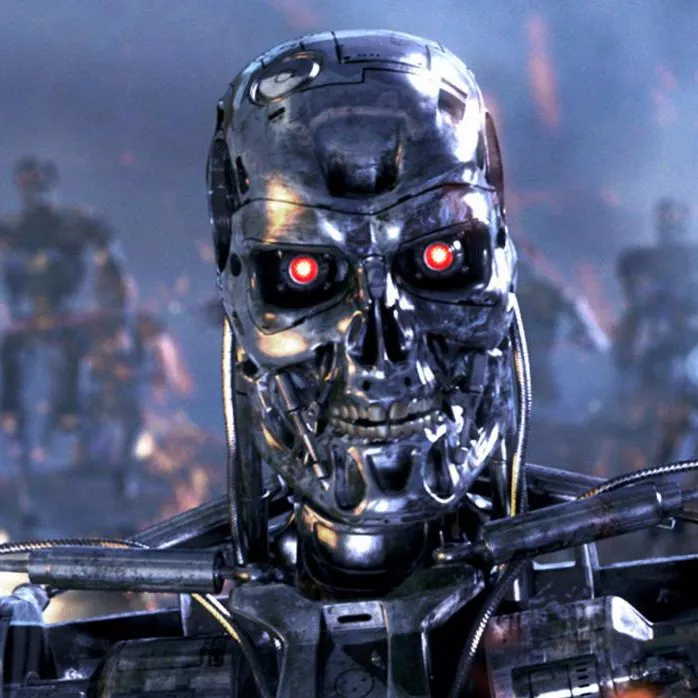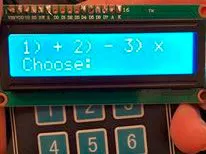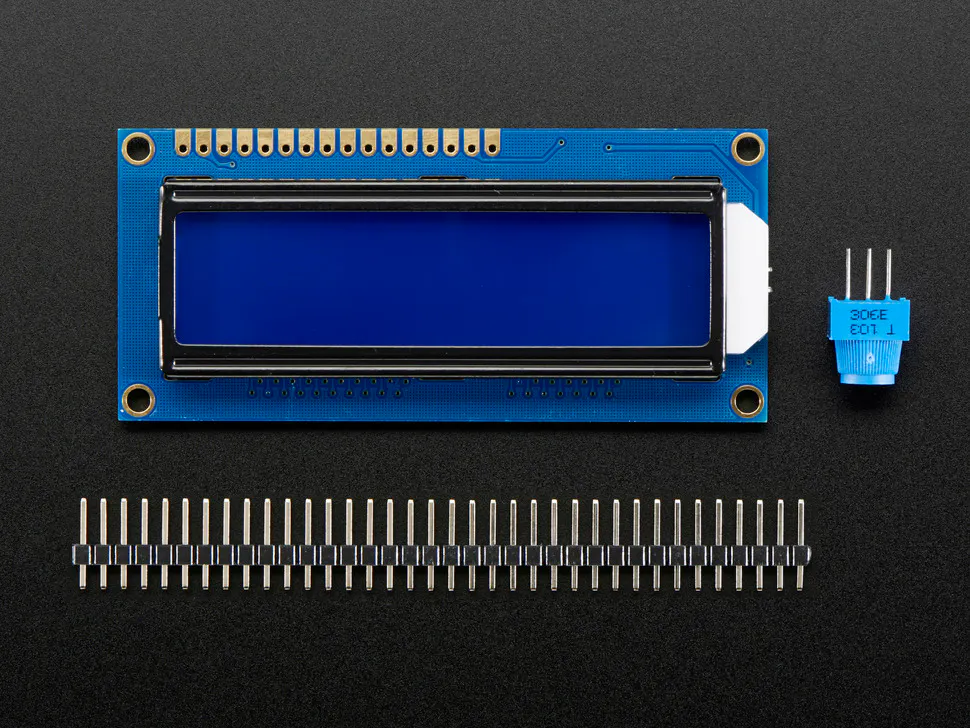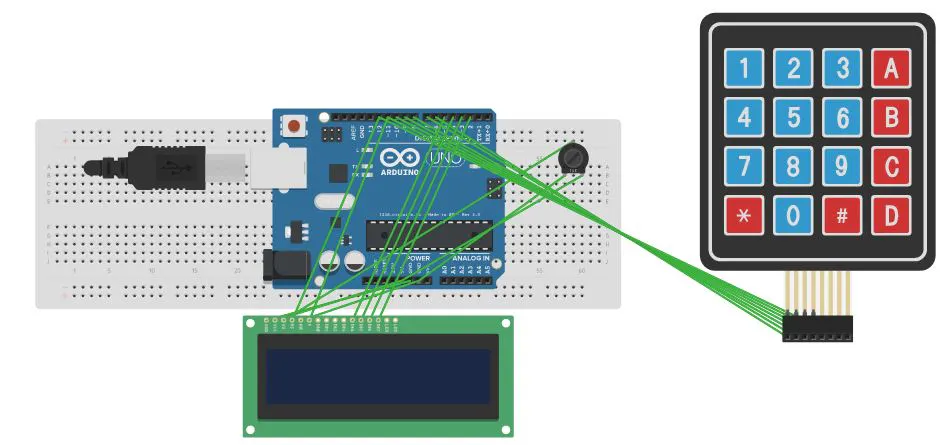Published

# Quizmo

IntermediateShowcase (no instructions)6 hours1,588## Things used in this project

### Hardware componentsArduino UNO & Genuino UNO
×1Adafruit Standard LCD - 16x2 White on Blue
×1
×1

### Software apps and online servicesArduino IDE

## Schematics

### Block Diagram

This is how whole system must look like## Code

### Arduino Code

C/C++
Upload this code directly to UNO and make sure you use the wiring similar to mentioned in code
```#include <Keypad.h>
#include <LiquidCrystal.h>

LiquidCrystal lcd(12, 11, 5, 4, 3, 2);

long rand1,rand2,ans,ans1,opr,score,total;
char selec,selec1;
boolean quiz=false;
String stringTwo;

const byte ROWS = 4; //four rows
const byte COLS = 3; //three columns
char keys[ROWS][COLS] = {
{'1','2','3'},
{'4','5','6'},
{'7','8','9'},
{'*','0','#'}
};
byte rowPins[ROWS] = {10, 9, 8, 7}; //connect to the row pinouts of the keypad
byte colPins[COLS] = {6,1,0}; //connect to the column pinouts of the keypad

void setup(){
lcd.begin(16, 2);
}

void loop(){
while (selec==NO_KEY){
}
lcd.print(selec);
delay(500);
if (selec!=NO_KEY){
switch(selec){
case '1':
quiz=true;
opr=1;
quiz_sum();
break;
case '2':
quiz=true;
opr=2;
quiz_subt();
break;
case '3':
quiz=true;
opr=3;
quiz_mult();
break;
}
}
}

lcd.clear();
lcd.setCursor(0, 0);
lcd.print("1)+ 2)- 3)x");
lcd.setCursor(0, 1);
lcd.print("Choose: ");
}
void quiz_sum(){
lcd.clear();
lcd.setCursor(0, 0);
rand1=random(1,99);
rand2=random(1,99);
lcd.print("Find ");
lcd.print(rand1);
lcd.print("+");
lcd.print(rand2);
lcd.print("?");
ans1=rand1+rand2;
while (selec1==NO_KEY){
}
while (quiz){
while (selec1==NO_KEY){
}
switch(selec1){
case NO_KEY:
break;
default:
lcd.print(selec1);
stringTwo.concat(selec1);
ans=stringTwo.toInt();
//lcd.print(stringTwo);
selec1=NO_KEY;
break;
}
}
}

void quiz_subt(){
lcd.clear();
lcd.setCursor(0, 0);
rand1=random(1,99);
rand2=random(1,99);
lcd.print("Find ");
if(rand1>=rand2){
lcd.print(rand1);
lcd.print("-");
lcd.print(rand2);
ans1=rand1-rand2;
}
else if(rand1<rand2){
lcd.print(rand2);
lcd.print("-");
lcd.print(rand1);
ans1=rand2-rand1;
}
lcd.print("?");
while (selec1==NO_KEY){
}
while (quiz){
while (selec1==NO_KEY){
}
switch(selec1){
case NO_KEY:
break;
default:
lcd.print(selec1);
stringTwo.concat(selec1);
ans=stringTwo.toInt();
//lcd.print(stringTwo);
selec1=NO_KEY;
break;
}
}
}

void quiz_mult(){
lcd.clear();
lcd.setCursor(0, 0);
rand1=random(1,12);
rand2=random(1,12);
lcd.print("Find ");
lcd.print(rand1);
lcd.print("x");
lcd.print(rand2);
lcd.print("?");
ans1=rand1*rand2;
while (selec1==NO_KEY){
}
while (quiz){
while (selec1==NO_KEY){
}
switch(selec1){
case NO_KEY:
break;
default:
lcd.print(selec1);
stringTwo.concat(selec1);
ans=stringTwo.toInt();
//lcd.print(stringTwo);
selec1=NO_KEY;
break;
}
}
}

void chk_ans(){
lcd.clear();
if (ans1==ans){
lcd.clear();
lcd.print("Correct");
score=score+1;
}
else {
lcd.clear();
lcd.print("Incorrect");
}
}
case PRESSED:
if (key == '*') {
chk_ans();
total=total+1;
stringTwo="";
delay(400);
if(opr==1){
quiz_sum();
}
else if(opr==2){
quiz_subt();
}
else if(opr==3){
quiz_mult();
}
}
else if (key == '#') {
quiz=false;
stringTwo="";
lcd.clear();
lcd.print(score);
lcd.print("/");
lcd.print(total);
delay(1500);
score=0;
total=0;
loop();
}
break;
}
}
```

## Credits

### Zeeshan

11 projects • 42 followers
Creative and destructive design master. Ability to use the brain waves for both construction and destruction